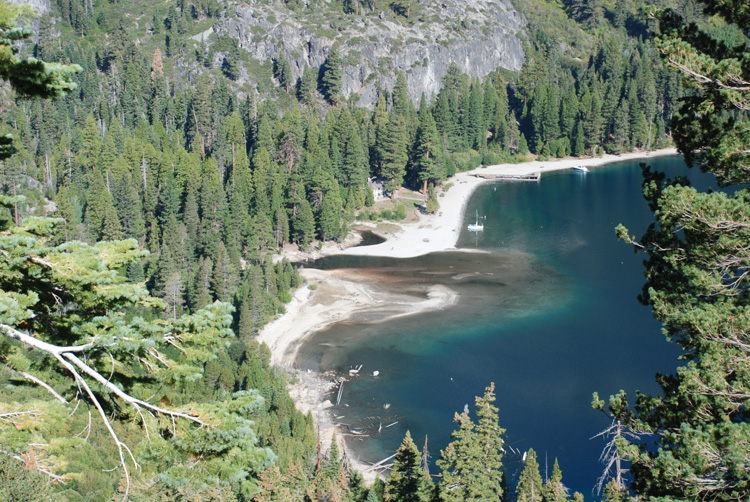There is a delta at the upper end of Emerald Bay where Eagle Creek empties into the bay. A very rough back of the envelope calculation of the erosion rate for this basin can be figured by calculating the volume of the delta, the drainage area, and the time elapsed since glaciation. The volume of the delta would be 300m x 300m x 20m = 1.8e6 m3. The drainage area is 3000m x 4000m = 1.2e7. The time elapsed since glaciation is 1e4 years. So the erosion rate would be 1.8e6 m3 / (1.2e7 m2 * 1e4 years) * 1000mm/m = 0.015 mm/year. A typical erosion rate (for any landscape) would be 0.1 mm/year, so as we walk up into the basin, we will try to figure out why this delta is so small.